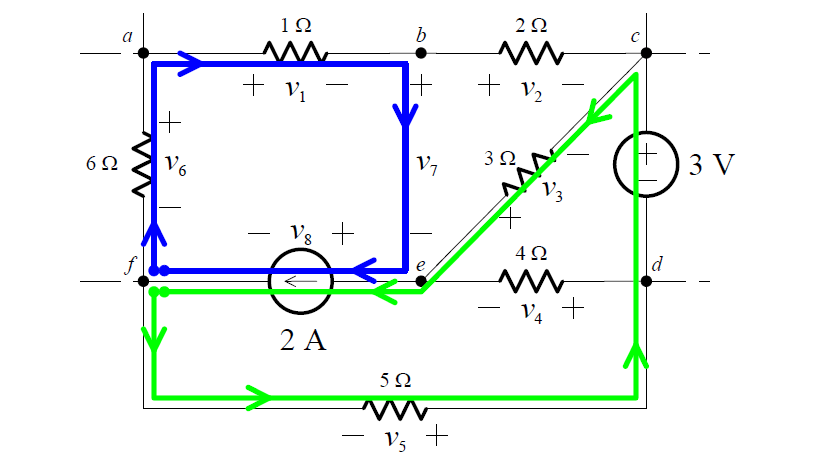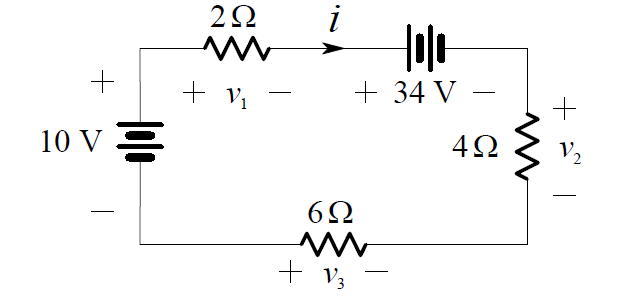# What is Kirchhoff’s Voltage Law

Starting at any node in a circuit, we form a loop by traversing through elements (open-circuits included!) and returning to the starting node, never encountering any other node more than once.

Figure 1 : For example, the paths fabef and fdcef are loops:Figure 2 : whereas the paths becba and fde are notKirchhoff’s  Voltage  Law  (KVL)  is  essentially  the  law  of  conservation  of energy. If voltages across elements traversed from – to + are positive in sense, and voltages across elements that are traversed from + to – are negative in sense (or vice versa), then KVL can be stated as follows:

KVL defined as

KVL: Around any loop in a circuit, the voltages algebraically sum to zero.

If there are n elements in the loop then, in symbols, KVL is:KVL can also be stated as: In traversing a loop, the sum of the voltage rises equals the sum of the voltage drops.

Example 1

In the circuit shown in Figure 1, we select a traversal from – to + to be positive in sense. Then KVL around the loop abcefa gives:

– v1 – v2  + v3  – v8  + v6  = 0

and around loop bcdeb, we have:

– v2  – 3 – v4  + v7  = 0

In this last loop, one of the elements traversed (the element between nodes b and e) is an open-circuit; however, KVL holds regardless of the nature of the elements in the circuit.

Example 2

We want to find the current i, in the one-loop circuit shown below:The polarities of v1 , v2 , v3 and the direction of i were chosen arbitrarily (the polarities of the 10 V and 34 V sources are given). Applying KVL we get:

10 – v1 – 34 – v2  + v3  = 0

Thus:

v1 + v2  – v3  = -24

From Ohm’s Law:

v1  = 2i

v2  = 4i

v3  = -6i

Substituting these into the previous equation yields:

(2i) + (4i) – (- 6i) = -24

2i + 4i + 6i = -24

12i = -24

i = -2 A

Having solved for i, we now find that:

v1  = 2i = 2(- 2) = -4 V

v2  = 4i = 4(- 2) = -8 V

v3  = -6i = (- 6)(- 2) = 12 V